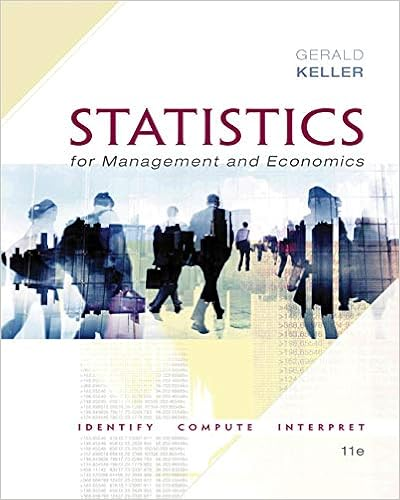# Using the command statdisplay descriptive statistics

• Lab Report
• 8

This preview shows page 4 - 6 out of 8 pages.

##### We have textbook solutions for you!
The document you are viewing contains questions related to this textbook.The document you are viewing contains questions related to this textbook.
Chapter 4 / Exercise 4.136
Statistics for Management and Economics + XLSTAT Bind-in
KellerExpert Verified
3. Using the command STAT>DISPLAY DESCRIPTIVE STATISTICS, determine the mean and standard deviation for each of the five groups. Paste the results here. Summary for n=1 Summary for n=30 Summary for n=30 Summary for n=30 Summary for n=1 Summary for n=5 Summary for n=10 Summary for n=30 Summary for n=100 Descriptive Statistics: CLT for mean, CLT for mean, CLT for mean, ... Variable N N* Mean SE Mean StDev Minimum Q1 Median
##### We have textbook solutions for you!
The document you are viewing contains questions related to this textbook.The document you are viewing contains questions related to this textbook.
Chapter 4 / Exercise 4.136
Statistics for Management and Economics + XLSTAT Bind-in
KellerExpert Verified
CLT for mean n=1 1000 0 97.66 3.24 102.37 0.01 28.39 65.88 CLT for mean n=5 1000 0 100.10 1.40 44.42 14.48 66.11 94.30 CLT for mean n=10 1000 0 100.01 0.986 31.19 20.31 77.33 98.98 CLT for mean n=30 1000 0 100.29 0.571 18.07 53.27 87.52 98.84 CLT for mean n=100 1000 0 100.34 0.324 10.24 68.75 93.62 100.11 Variable Q3 Maximum CLT for mean n=1 132.79 733.42 CLT for mean n=5 123.91 274.72 CLT for mean n=10 118.91 240.10 CLT for mean n=30 112.38 168.62 CLT for mean n=100 106.21 136.17
b. As the sample size increases, describe the change in standard deviation.
4. What you have observed are the three important parts of the Central Limit Theorem for the distribution of the sample mean In your own words, describe these three important parts.
•••